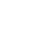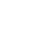August 14, 20233 minute read# Parallel Computing on Dagster with DaskName
Odette Harary
Handle
@odetteDask (https://www.dask.org/) is a flexible library for parallel computing in Python which can be a helpful tool when performing larger data engineering and machine learning tasks. Let's review how to use Dagster’s resources to automate your Dask computations and make your pipelines faster.

In this guide, you’ll learn how to:

## Using Dagster’s resources to set up a Dask Cluster

When using Dask with Dagster, you can define a `dask_resource` to set up a Dask client to share Dask configuration across multiple assets and simplify a pipeline. In this example, a local `dask.distributed` client is set up as a `ConfigurableResource`. Resources allow you to centralize your configuration in a single location, reducing redundant code in your assets.

``````from dask.distributed import Client
from dagster import ConfigurableResource

client = Client()
return client
``````

The `dask.distributed.LocalCluster` API allows customization of the cluster, which can be leveraged through Dagster’s resource. In the following example, configuration is setup at the resource level, which is then leveraged by `Definitions` and applied to all uses of the resource.

``````class dask_resource(ConfigurableResource):
n_workers : int

client = Client(LocalCluster(n_workers=self.n_workers))
return client

defs = Definitions(
assets=[asset_1, asset_2],
``````

In the following example, the `LocalCluster` configuration is being used in `make_dask_cluster`, which can be set for each `@asset` .

``````class dask_resource(ConfigurableResource):

client = Client(LocalCluster(n_workers))
return client

@asset
``````

Now that the Dask resource is set up, an asset can use it to parallelize computations. This asset demonstrates using the `client` within Dagster using the example from the Dask distributed quickstart.

``````from dagster import asset, MetadataValue
@asset
def square(x):
return x ** 2
def neg(x):
return -x
A = client.map(square, range(10000))
B = client.map(neg, A)
total = client.submit(sum, B)

defs = Definitions(
``````

Note: The Dask UI, which can accessed at http://localhost:8787/status by default, or using `client.dashboard_link` can be used to monitor the executions from Dagster which can be accessed through the link generated in the Dagster metadata. The Dask UI is only available while executions are in progress since Dagster is only spinning up the resource when the asset needs it.

Which can be viewed executing in the Dask UI:

## Speeding up processes using Dask

One of the benefits of using Dask with Dagster is speed. In the next example, we modified the `hackernews_stories` asset from Building machine learning pipelines with Dagster to distribute the API call for Hacker News.

``````from dagster import asset, MetadataValue
import requests
import pandas as pd

@asset
latest_item = requests.get(
"https://hacker-news.firebaseio.com/v0/maxitem.json"
).json()
results = []
scope = range(latest_item - 1000, latest_item)

def get_single_line(item_id):
item = requests.get(
f"https://hacker-news.firebaseio.com/v0/item/{item_id}.json"
).json()
return item

for item_id in scope:
results.append(y)

df = pd.DataFrame(results)
client.close()
return df
``````

The Dask UI shows each instance of `get_single_line` being scheduled by Dask:

The Dagster UI can be used to review the execution timing. In this case, the original `hackernews_stories` takes almost two minutes to execute for 1,000 records whereas `hackernews_stories_dask` only takes about 18 seconds.

## Building a machine learning pipeline using Dask

Now that you walked through using the `dask_resource` for specific assets, next you’ll build a machine learning pipeline using Dask.

First, you can use `dask_ml.datasets` to generate some synthetic data using the `make_classification` API. Choosing the appropriate chunk size can help optimize the processing, this post give more insight into choosing chunk sizes. Choosing too small of a chunk size will produce more work for Dask to manage scheduling, while choosing a larger chunk size can limit Dask’s ability to distribute the work.

``````from dask_ml.datasets import make_classification

@asset
X, y = make_classification(chunks=200, n_samples=10000)
return X, y
``````

Next, use Dask’s method, `model_selection.train_test_split`, to generate a training and test set of data using Dagster’s `multi_asset`. Dask will produce the training and test sets as four `dask.arrays`.

``````from dagster import multi_asset, AssetOut

@multi_asset(outs={"training_data": AssetOut(), "test_data": AssetOut()})
X, y = my_classification_data
X_train, X_test, y_train, y_test= model_selection.train_test_split(X, y)
return (X_train, y_train), (X_test, y_test)
``````

Now that you have `X_train`,`X_test`,`y_train`,`y_test`, you can search for the best classification model using sklearn’s `SGDClassifier` and Dask’s `HyperbandSearchCV`.

`SGDClassifier` uses an iterative training algorithm that runs multiple iterations to decrease loss or how poorly the model predicted a value. Many parameters can be used for the `SGDClassifier`, so rather than checking each of the combinations manually to see what the best results are, hyper-parameter tuning can be used.

Hyperparameter tuning searches through a range of different models and parameter values to find the best one for your data. In this example, Dask’s `HyperbandSearchCV` is being used to test each of the combinations defined in the `param_dist`. `HyperbandSearchCV` finds the best parameters by focusing on high-performing estimators versus a more random search algorithm that might try random parameters.

The parameters of the best model can be stored as Dagster `metadata` to view in the Dagster UI. The model is returned, which can be used for inference or predictions.

``````from sklearn.linear_model import SGDClassifier
import numpy as np

@asset
X_train, y_train = training_data
est = SGDClassifier(tol=1e-3)
param_dist = {'alpha': np.logspace(-4, 0, num=1000),
'loss': ['hinge', 'log', 'modified_huber', 'squared_hinge'],
'average': [True, False]}
search = HyperbandSearchCV(est, param_dist)
search.fit(X_train, y_train, classes=np.unique(y_train))
model = search.best_estimator_
return model
``````

The `loss`, `average`, and `alpha` parameters from `search.best_params_` are available in the Dagster UI.

The Dask dashboard is available while the execution is in progress and shows the tasks submitted to the Dask resource.

The last asset in the pipeline will use `test_data` to score the model. This can also be done in the model asset or separately, depending on your specific machine learning use case.

``````@asset
X_test, y_test = test_data
my_score = my_model.score(X_test, y_test)
return my_score
``````

The final step is loading the assets into a code location or `Definitions`. The `dask_resource` is included with the `assets`.

``````from dagster import Definitions

defs = Definitions(
assets=[my_classification_data, train_test_data, my_model, my_score ],
``````

Great job setting up your Dask resource and building a machine learning pipeline using Dask in Dagster!

We're always happy to hear your feedback, so please reach out to us! If you have any questions, ask them in the Dagster community Slack (join here!) or start a Github discussion. If you run into any bugs, let us know with a Github issue. And if you're interested in working with us, check out our open roles!Integration